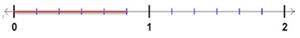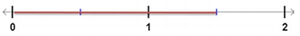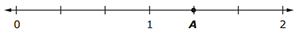# MAFS.3.NF.1.2

Understand a fraction as a number on the number line; represent fractions on a number line diagram.
1. Represent a fraction 1/b on a number line diagram by defining the interval from 0 to 1 as the whole and partitioning it into b equal parts. Recognize that each part has size 1/b and that the endpoint of the part based at 0 locates the number 1/b on the number line.
2. Represent a fraction a/b on a number line diagram by marking off a lengths 1/b from 0. Recognize that the resulting interval has size a/b and that its endpoint locates the number a/b on the number line.

### Clarifications

Example of Opportunities for In-Depth Focus

Developing an understanding of fractions as numbers is essential for future work with the number system. It is critical that students at this grade are able to place fractions on a number line diagram and understand them as a related component of their ever- expanding number system.

Fluency Expectations or Examples of Culminating Standards

Students fluently add and subtract within 1000 using strategies and algorithms based on place value, properties of operations, and/or the relationship between addition and subtraction. 3.NBT.1.2 a relatively small and incremental expectation.
General Information
Subject Area: Mathematics
Domain-Subdomain: Number and Operations - Fractions
Cluster: Level 2: Basic Application of Skills & Concepts
Cluster: Develop understanding of fractions as numbers. (Major Cluster) -

Clusters should not be sorted from Major to Supporting and then taught in that order. To do so would strip the coherence of the mathematical ideas and miss the opportunity to enhance the major work of the grade with the supporting clusters.

Date of Last Rating: 02/14
Status: State Board Approved
Assessed: Yes
Test Item Specifications

• Assessment Limits :
Denominators are limited to 2, 3, 4, 6, and 8. Number lines in MAFS.3.NF.1.2b items may extend beyond 1. Only whole number marks may be labeled on number lines.
• Calculator :

No

• Context :

No context

Sample Test Items (4)

• Test Item #: Sample Item 2
• Question:

Which fraction is represented by the total length marked on the number line shown?• Difficulty: N/A
• Type: EE: Equation Editor

• Test Item #: Sample Item 3
• Question:

What fraction is represented by the length marked on the number line shown?• Difficulty: N/A
• Type: EE: Equation Editor

• Test Item #: Sample Item 4
• Question:

Only for MAFS.3.NF.1.2b:

What fraction is represented by point A on the number line shown?• Difficulty: N/A
• Type: EE: Equation Editor

## Related Courses

This benchmark is part of these courses.
5012050: Grade Three Mathematics (Specifically in versions: 2014 - 2015, 2015 - 2022 (current), 2022 and beyond)
7712040: Access Mathematics Grade 3 (Specifically in versions: 2014 - 2015, 2015 - 2018, 2018 - 2022 (current), 2022 and beyond)
5012055: Grade 3 Accelerated Mathematics (Specifically in versions: 2019 - 2022 (current), 2022 and beyond)
5012015: Foundational Skills in Mathematics 3-5 (Specifically in versions: 2019 - 2022 (current), 2022 and beyond)

## Related Access Points

Alternate version of this benchmark for students with significant cognitive disabilities.
MAFS.3.NF.1.AP.2a: Locate given common unit fractions (i.e., 1/2, 1/4) on a number line or ruler.

## Related Resources

Vetted resources educators can use to teach the concepts and skills in this benchmark.

## Assessments

Sample 4 - Third Grade Math State Interim Assessment:

This is a State Interim Assessment for third grade.

Type: Assessment

Sample 3 - Third Grade Math State Interim Assessment:

This is a State Interim Assessment for third grade.

Type: Assessment

Sample 2 - Third Grade Math State Interim Assessment:

This is a State Interim Assessment for third grade.

Type: Assessment

## Formative Assessments

Four-Sixths on the Number Line:

Students are asked to use a number line that includes the location of zero and one-sixth to find the location of four-sixths.

Type: Formative Assessment

One-Third on the Number Line:

Students are given four number line diagrams and asked to choose the one that correctly shows the location of one-third.

Type: Formative Assessment

Five-Eighths on the Number Line:

Students are asked to locate five-eighths on a number line that has been anchored by zero and one, but that has not yet been scaled.

Type: Formative Assessment

Three-Fourths on the Number Line:

Students are asked to scale a number line from zero to one so that they can find the location of three-fourths.

Type: Formative Assessment

## Lesson Plans

Fraction Flyers :

Students will represent fractions between zero and one by completing a number line of their own. Students will use their understanding of fractions between zero and one to design their own number line. Students will then communicate their understanding of representing fractions on a number line through a written proposal.

Type: Lesson Plan

Discovering Fractions:

In this lesson students will make initial discoveries about fractions. Students will work together to explain and record the discoveries they make while using manipulatives to explore fractions.

Type: Lesson Plan

The Human Number Line:

This lesson uses a human number line to help students estimate a fraction's approximate position on the number line between zero and one. It also helps students visualize and understand the relative size of fractions, preparing them to be able to make comparisons.

Type: Lesson Plan

Fraction Counting Book:

Students will make a book of fractions by counting by a specified denominator. They will represent the fractions as pictures, in number form, word form, and on a number line.

Type: Lesson Plan

Interactive Fraction Number Lines:

In this lesson students make models of fractions, including a human number line. Using a number line, students develop conceptual understanding of fractions. Students use the number line to represent and compare fractions less than one. The activities are engaging and include full participation/engagement of all students. The fractions are limited to positive fractions less than one with a denominator of 2 or 4 including 0 and 1 whole.

Type: Lesson Plan

Fractions on a Number line:

In this lesson, students will have experiences with determining where a given fraction falls in regards to "benchmark" fractions and will practice placing fractions on a number line.

Type: Lesson Plan

The Fraction String:

In this lesson students create a model of a number line using string and adding machine tape. Students discover how to partition the string into equal sections, and name the fractional pieces, including fractions greater than 1.

Type: Lesson Plan

## Lesson Study Resource Kit

Number and Operations - Fractions Lesson Study Resource Kit – Third Grade:

This lesson study resource kit can be used to guide and support teams of third grade teachers as they engage in lesson study focused on the academic standards in the Number and Operations - Fractions domain.

Type: Lesson Study Resource Kit

## Original Student Tutorials

Fraction Camp! Fractions Greater Than 1 on a Number Line:

Joey uses his knowledge of fractions to win games at camp by knowing where fractions greater than one are located on number lines, in this interactive tutorial.

Type: Original Student Tutorial

Nature Walk: Fractions Less Than 1 on a Number Line:

Learn to use number lines to represent fractions as Emmy explores nature in this interactive tutorial.

Type: Original Student Tutorial

Finding Fractions at Camp: Fractions on a Number Line:

Joey learns about the location of unit fractions on a number line while at camp in this interactive tutorial.

Type: Original Student Tutorial

Find 7/4 starting from 1, Assessment Variation:

Part (a) of the standard is about representing unit fractions and part (b) is about representing fractions in terms of unit fractions. The tasks require attention to the whole when thinking about fractions; on a number line, the whole is the interval from 0 to 1.

Find 1/4 Starting from 1, Assessment Version:

Part (a) of the standard is about representing unit fractions and part (b) is about representing fractions in terms of unit fractions. Each requires that students "understand a fraction as a number on the number line" and "represent fractions on a number line diagram."

Find 1 Starting from 5/3, Assessment Variation:

Part (a) of the standard is about representing unit fractions and part (b) is about representing fractions in terms of unit fractions. Each requires that students "understand a fraction as a number on the number line" and "represent fractions on a number line diagram."

Closest to 1/2:

How students tackle the problem and the amount of work they show on the number line can provide insight into the sophistication of their thinking. As students partition the interval between 0 and 1 into eighths, they will need to recognize that 1/2=4/8. Students who systematically plot every point, even 9/8, which is larger even than 1 may still be coming to grips with the relative size of fractions.

Locating Fractions Greater than One on the Number Line:

The goal of this task is to help students gain a better understanding of fractions and their place on the number line.

Find 2/3:

This simple-looking problem reveals much about how well students understand unit fractions as well as representing fractions on a number lin

Find 1:

This task includes the seeds of several important ideas. Part a presents the student with the opportunity to use a unit fraction to find 1 on the number line, a critical aspect for meeting standard 3.NF.2b. Part b helps reinforce the notion that when a fraction has a numerator that is larger than the denominator, it has a value greater than 1 on the number line.

Which is Closer to 1?:

The purpose of this task is for students to identify which fraction is closest to the whole number 1.

Locating Fractions Less than One on the Number Line:

In every part of this task, students must treat the interval from 0 to 1 as a whole, partition the whole into the appropriate number of equal sized parts, and then locate the fraction(s).

## Student Center Activity

Students can practice answering mathematics questions on a variety of topics. With an account, students can save their work and send it to their teacher when complete.

Type: Student Center Activity

## Virtual Manipulative

Fraction Game:

This virtual manipulative allows individual students to work with fraction relationships. (There is also a link to a two-player version.)

Type: Virtual Manipulative

## MFAS Formative Assessments

Five-Eighths on the Number Line:

Students are asked to locate five-eighths on a number line that has been anchored by zero and one, but that has not yet been scaled.

Four-Sixths on the Number Line:

Students are asked to use a number line that includes the location of zero and one-sixth to find the location of four-sixths.

One-Third on the Number Line:

Students are given four number line diagrams and asked to choose the one that correctly shows the location of one-third.

Three-Fourths on the Number Line:

Students are asked to scale a number line from zero to one so that they can find the location of three-fourths.

## Original Student Tutorials Mathematics - Grades K-5

Finding Fractions at Camp: Fractions on a Number Line:

Joey learns about the location of unit fractions on a number line while at camp in this interactive tutorial.

Fraction Camp! Fractions Greater Than 1 on a Number Line:

Joey uses his knowledge of fractions to win games at camp by knowing where fractions greater than one are located on number lines, in this interactive tutorial.

Nature Walk: Fractions Less Than 1 on a Number Line:

Learn to use number lines to represent fractions as Emmy explores nature in this interactive tutorial.

## Student Resources

Vetted resources students can use to learn the concepts and skills in this benchmark.

## Original Student Tutorials

Fraction Camp! Fractions Greater Than 1 on a Number Line:

Joey uses his knowledge of fractions to win games at camp by knowing where fractions greater than one are located on number lines, in this interactive tutorial.

Type: Original Student Tutorial

Nature Walk: Fractions Less Than 1 on a Number Line:

Learn to use number lines to represent fractions as Emmy explores nature in this interactive tutorial.

Type: Original Student Tutorial

Finding Fractions at Camp: Fractions on a Number Line:

Joey learns about the location of unit fractions on a number line while at camp in this interactive tutorial.

Type: Original Student Tutorial

Find 7/4 starting from 1, Assessment Variation:

Part (a) of the standard is about representing unit fractions and part (b) is about representing fractions in terms of unit fractions. The tasks require attention to the whole when thinking about fractions; on a number line, the whole is the interval from 0 to 1.

Find 1 Starting from 5/3, Assessment Variation:

Part (a) of the standard is about representing unit fractions and part (b) is about representing fractions in terms of unit fractions. Each requires that students "understand a fraction as a number on the number line" and "represent fractions on a number line diagram."

Closest to 1/2:

How students tackle the problem and the amount of work they show on the number line can provide insight into the sophistication of their thinking. As students partition the interval between 0 and 1 into eighths, they will need to recognize that 1/2=4/8. Students who systematically plot every point, even 9/8, which is larger even than 1 may still be coming to grips with the relative size of fractions.

Locating Fractions Greater than One on the Number Line:

The goal of this task is to help students gain a better understanding of fractions and their place on the number line.

Find 2/3:

This simple-looking problem reveals much about how well students understand unit fractions as well as representing fractions on a number lin

Find 1:

This task includes the seeds of several important ideas. Part a presents the student with the opportunity to use a unit fraction to find 1 on the number line, a critical aspect for meeting standard 3.NF.2b. Part b helps reinforce the notion that when a fraction has a numerator that is larger than the denominator, it has a value greater than 1 on the number line.

Which is Closer to 1?:

The purpose of this task is for students to identify which fraction is closest to the whole number 1.

Locating Fractions Less than One on the Number Line:

In every part of this task, students must treat the interval from 0 to 1 as a whole, partition the whole into the appropriate number of equal sized parts, and then locate the fraction(s).

## Student Center Activity

Students can practice answering mathematics questions on a variety of topics. With an account, students can save their work and send it to their teacher when complete.

Type: Student Center Activity

## Virtual Manipulative

Fraction Game:

This virtual manipulative allows individual students to work with fraction relationships. (There is also a link to a two-player version.)

Type: Virtual Manipulative

## Parent Resources

Vetted resources caregivers can use to help students learn the concepts and skills in this benchmark.

Find 7/4 starting from 1, Assessment Variation:

Part (a) of the standard is about representing unit fractions and part (b) is about representing fractions in terms of unit fractions. The tasks require attention to the whole when thinking about fractions; on a number line, the whole is the interval from 0 to 1.

Find 1/4 Starting from 1, Assessment Version:

Part (a) of the standard is about representing unit fractions and part (b) is about representing fractions in terms of unit fractions. Each requires that students "understand a fraction as a number on the number line" and "represent fractions on a number line diagram."

Find 1 Starting from 5/3, Assessment Variation:

Part (a) of the standard is about representing unit fractions and part (b) is about representing fractions in terms of unit fractions. Each requires that students "understand a fraction as a number on the number line" and "represent fractions on a number line diagram."

Closest to 1/2:

How students tackle the problem and the amount of work they show on the number line can provide insight into the sophistication of their thinking. As students partition the interval between 0 and 1 into eighths, they will need to recognize that 1/2=4/8. Students who systematically plot every point, even 9/8, which is larger even than 1 may still be coming to grips with the relative size of fractions.

Locating Fractions Greater than One on the Number Line:

The goal of this task is to help students gain a better understanding of fractions and their place on the number line.

Find 2/3:

This simple-looking problem reveals much about how well students understand unit fractions as well as representing fractions on a number lin

Find 1:

This task includes the seeds of several important ideas. Part a presents the student with the opportunity to use a unit fraction to find 1 on the number line, a critical aspect for meeting standard 3.NF.2b. Part b helps reinforce the notion that when a fraction has a numerator that is larger than the denominator, it has a value greater than 1 on the number line.